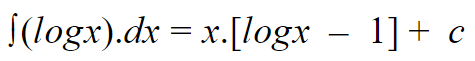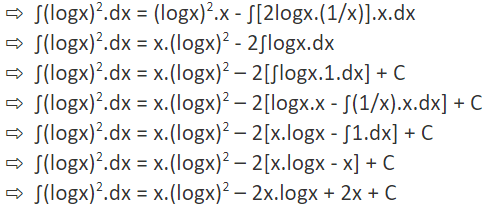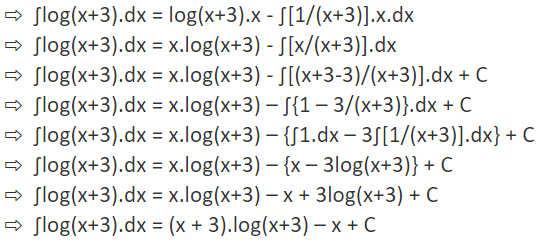Maths
Study Material

# Integration of log x

The integration of log x is calculated by the technique (or method) of Integration by Parts (also known as Partial Integration or Product Rule of Integration).

4 minutes long
Posted by Kunduz Tutor, 3/11/2021Hesap Oluştur

Got stuck on homework? Get your step-by-step solutions from real tutors in minutes! 24/7. Unlimited.

## What is Integration of log x ?

The integration of log x is given as:The integration of log x is calculated by the technique (or method) of Integration by Parts (also known as Partial Integration or Product Rule of Integration).

Author – Ojasvi Chaplot

## Derivation

By using the formula of integration by parts or by the method of product rule of integration, we will obtain the log x integration.

As we know that the formula of integration by parts is:

∫f(x)g(x).dx = f(x)∫g(x).dx − ∫(f′(x)∫g(x).dx).dx + C

And, we can write logx as (logx.1)

So, ? ∫logx.dx = ∫logx.1.dx

Now,

By using the ILATE Rule,

Let, f(x) = logx and g(x) = 1.

? f’(x) = 1/x.

And,
? ∫g(x).dx = ∫1.dx
? ∫g(x).dx = x.
Then,
By using integration by parts,

=> ∫logx.dx = logx.x – ∫(1/x).x.dx
? ∫logx.dx = x.logx – ∫1.dx
=> ∫logx.dx = x.logx – x + C
? ∫logx.dx = x.(logx – 1) + C
Therefore,
Integration of Log x is ∫logx.dx = x.(logx – 1) + C ; where C = Integration constant.

## Examples using Integration of log x

Let’s take a look at some more illustrative examples which consist of logx along with some modifications and variations.

#### Example-1: Solve: ∫log(x/10).dx

Solution:
By using the formula of integration by parts, we will obtain the integration of log(x/10).
As we know that the formula of integration by parts is:
∫f(x)g(x).dx = f(x)∫g(x).dx − ∫(f′(x)∫g(x).dx).dx + C
And, we can write log(x/10) as:
? log(x/10) = [log(x/10).1]
So,
? ∫log(x/10).dx = ∫log(x/10).1.dx
Now,
By using the ILATE Rule,
Let, f(x) = log(x/10) and g(x) = 1.
? f’(x) = [1/(x/10)].(1/10)
? f’(x) = 1/x.
And,
? ∫g(x).dx = ∫1.dx
? ∫g(x).dx = x.
Then,
By using integration by parts,
? ∫log(x/10).dx = log(x/10).x – ∫(1/x).x.dx
=>∫log(x/10).dx = x.log(x/10) – ∫1.dx
? ∫log(x/10).dx = x.log(x/10) – x + C
=>∫log(x/10).dx = x.(log(x/10) – 1) + C
Therefore,
Answer: ∫log(x/10).dx = x.(log(x/10) – 1) + C ; where C = Integration constant.

#### Example-2: Find out the integral of x logx.

Solution:
By using the formula of integration by parts, we will calculate the integration of x logx.
As we know that the formula of integration by parts is:
∫f(x)g(x).dx = f(x)∫g(x).dx − ∫(f′(x)∫g(x).dx).dx + C
Now,
By using the ILATE Rule,
Let, f(x) = logx and g(x) = x.
? f’(x) = 1/x.
And,
? ∫g(x).dx = ∫x.dx
? ∫g(x).dx = x2/2.
Then,
By using integration by parts,
? ∫x.logx.dx = logx.(x2/2) – ∫(1/x).(x2/2).dx
=>∫x.logx.dx = (x2/2).logx – ∫(x/2).dx
? ∫x.logx.dx = (x2/2).logx – (1/2).(x2/2) + C
=>∫x.logx.dx = (x2/2).logx – (x2/4) + C
Hence, ∫x.logx.dx = (x2/2).logx – (x2/4) + C.
Answer: ∫x.logx.dx = (x2/2).logx – (x2/4) + C ; where C = Integration constant

#### Example-3: Calculate the integral of (logx)2.

Solution:
By using the formula of integration by parts, we will find out the integration of (logx)2.
As we know that the formula of integration by parts is:
∫f(x)g(x).dx = f(x)∫g(x).dx − ∫(f′(x)∫g(x).dx).dx + C
And, we can write (logx)2 as 1.(logx)2
So,
∫(logx)2.dx = ∫1.(logx)2dx

Now,
By using the integration by parts and ILATE Rule,Thus,
Answer: ∫(logx)2.dx = x.(logx)2 – 2x.logx + 2x + C ; where C = Integration constant.

#### Example-4: Solve the integration of log(x+1).

Solution:
By using the formula of integration by parts, we will obtain the integration of log(x+3).
As we know that the formula of integration by parts is:
∫f(x)g(x).dx = f(x)∫g(x).dx − ∫(f′(x)∫g(x).dx).dx + C
And, we can write log(x+3) as [log(x+3).1]
So,
∫log(x+3).dx = ∫[log(x+3).1].dx
Now,
By using the ILATE Rule,
Let, f(x) = log(x+3) and g(x) = 1.
? f’(x) = 1/(x+3).
And,
? ∫g(x).dx = ∫1.dx
? ∫g(x).dx = x.
Then,
By using integration by parts,Therefore,
Answer: ∫log(x+3).dx = (x + 3).log(x+3) – x + C ; where C = Integration constant.

## Picked For You

Furthermore, these are some topics which might interest you: